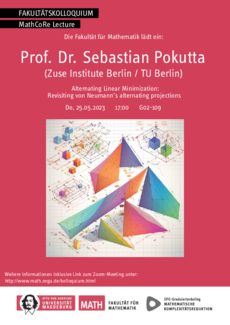## Prof. Dr. Sebastian PokuttaIn 1933 von Neumann proved a beautiful result that one can compute a point in the intersection of two convex sets (under suitable assumptions) by alternating projections, i.e., successively projecting on one set and then the other. This algorithm assumes that one has access to projection operators for both sets. Here we consider the much weaker setup where we have only access to linear minimization oracles over the convex sets and present an algorithm to find a point in the intersection of two convex sets. Moreover, we provide a modification of the algorithm to minimize a linear function over the intersection and discuss further extensions.

25.05.2023, Raum: G02-109, Zeit: 17:00

Letzte Änderung: 11.05.2023 - Ansprechpartner: Volker Kaibel